# Statistics Econometrics Statistics Econometrics Statistics Econometrics Statistics for

• Slides: 11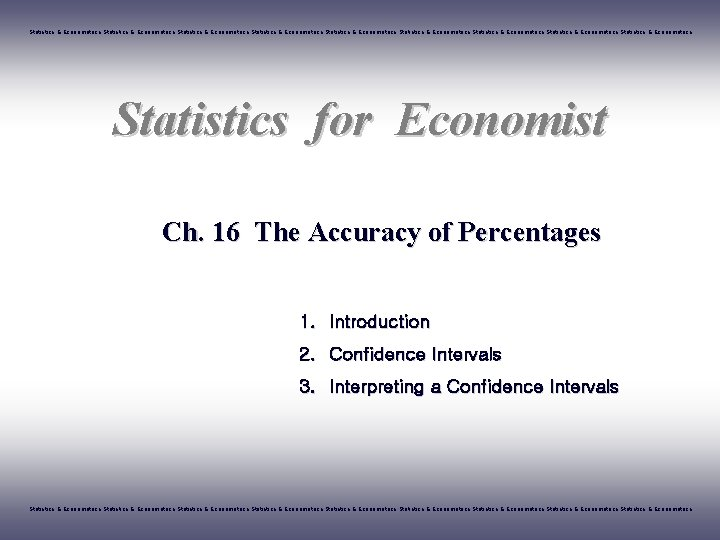Statistics & Econometrics Statistics & Econometrics Statistics & Econometrics Statistics for Economist Ch. 16 The Accuracy of Percentages 1. Introduction 2. Confidence Intervals 3. Interpreting a Confidence Intervals Statistics & Econometrics Statistics & Econometrics Statistics & Econometrics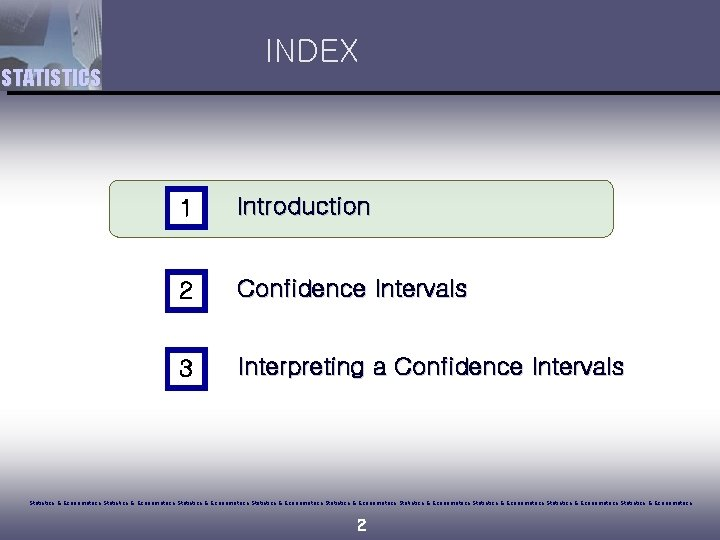INDEX STATISTICS 1 Introduction 2 Confidence Intervals 3 Interpreting a Confidence Intervals Statistics & Econometrics Statistics & Econometrics Statistics & Econometrics 2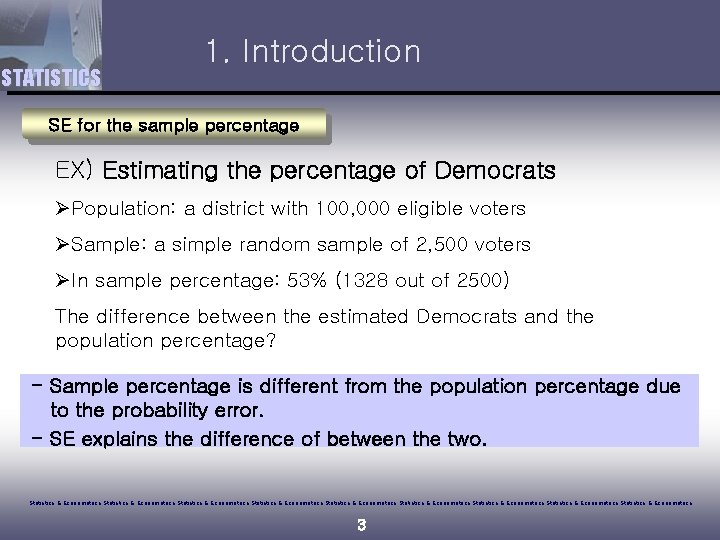STATISTICS 1. Introduction SE for the sample percentage EX) Estimating the percentage of Democrats ØPopulation: a district with 100, 000 eligible voters ØSample: a simple random sample of 2, 500 voters ØIn sample percentage: 53% (1328 out of 2500) The difference between the estimated Democrats and the population percentage? - Sample percentage is different from the population percentage due to the probability error. - SE explains the difference of between the two. Statistics & Econometrics Statistics & Econometrics Statistics & Econometrics 3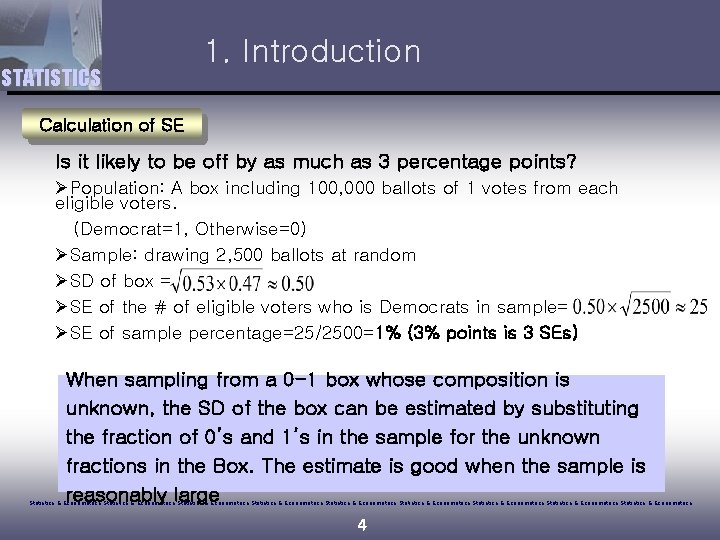STATISTICS 1. Introduction Calculation of SE Is it likely to be off by as much as 3 percentage points? ØPopulation: A box including 100, 000 ballots of 1 votes from each eligible voters. (Democrat=1, Otherwise=0) ØSample: drawing 2, 500 ballots at random ØSD of box = ØSE of the # of eligible voters who is Democrats in sample= ØSE of sample percentage=25/2500=1% (3% points is 3 SEs) When sampling from a 0 -1 box whose composition is unknown, the SD of the box can be estimated by substituting the fraction of 0’s and 1’s in the sample for the unknown fractions in the Box. The estimate is good when the sample is reasonably large Statistics & Econometrics Statistics & Econometrics Statistics & Econometrics 4INDEX STATISTICS 1 Introduction 2 Confidence Intervals 3 Interpreting a Confidence Intervals Statistics & Econometrics Statistics & Econometrics Statistics & Econometrics 5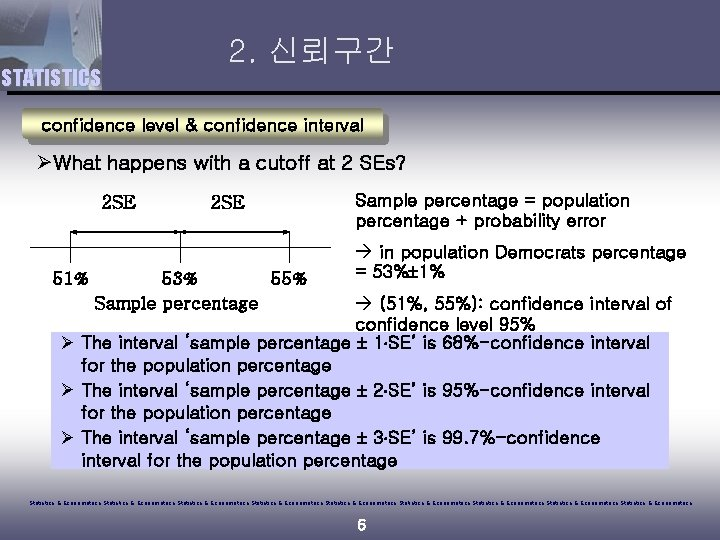STATISTICS 2. 신뢰구간 confidence level & confidence interval ØWhat happens with a cutoff at 2 SEs? 2 SE 51% 2 SE 53% 55% Sample percentage = population percentage + probability error à in population Democrats percentage = 53% 1% à (51%, 55%): confidence interval of confidence level 95% Ø The interval ‘sample percentage 1 SE’ is 68%-confidence interval for the population percentage Ø The interval ‘sample percentage 2 SE’ is 95%-confidence interval for the population percentage Ø The interval ‘sample percentage 3 SE’ is 99. 7%-confidence interval for the population percentage Statistics & Econometrics Statistics & Econometrics Statistics & Econometrics 6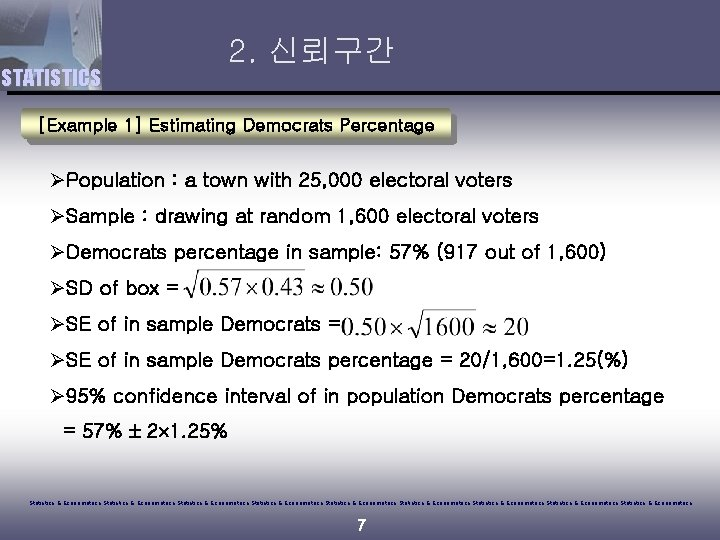STATISTICS 2. 신뢰구간 [Example 1] Estimating Democrats Percentage ØPopulation : a town with 25, 000 electoral voters ØSample : drawing at random 1, 600 electoral voters ØDemocrats percentage in sample: 57% (917 out of 1, 600) ØSD of box = ØSE of in sample Democrats percentage = 20/1, 600=1. 25(%) Ø 95% confidence interval of in population Democrats percentage = 57% 2 1. 25% Statistics & Econometrics Statistics & Econometrics Statistics & Econometrics 7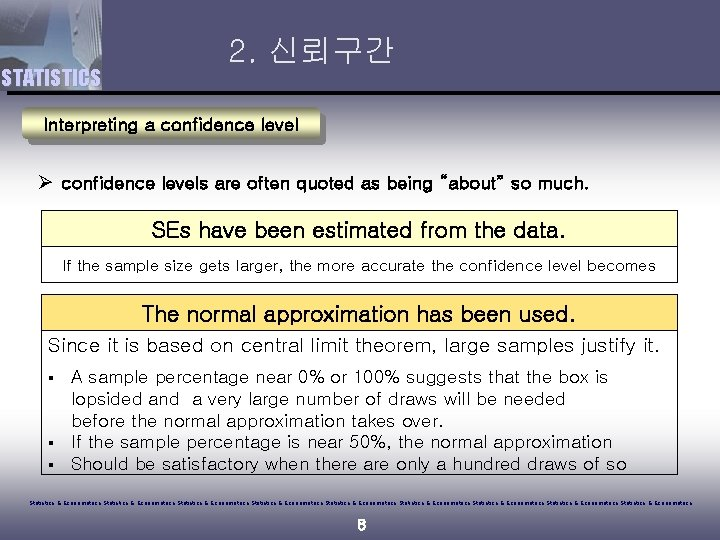STATISTICS 2. 신뢰구간 Interpreting a confidence level Ø confidence levels are often quoted as being “about” so much. SEs have been estimated from the data. If the sample size gets larger, the more accurate the confidence level becomes The normal approximation has been used. Since it is based on central limit theorem, large samples justify it. § § § A sample percentage near 0% or 100% suggests that the box is lopsided and a very large number of draws will be needed before the normal approximation takes over. If the sample percentage is near 50%, the normal approximation Should be satisfactory when there are only a hundred draws of so Statistics & Econometrics Statistics & Econometrics Statistics & Econometrics 8INDEX STATISTICS 1 Introduction 2 Confidence Intervals 3 Interpreting a Confidence Intervals Statistics & Econometrics Statistics & Econometrics Statistics & Econometrics 93. Interpreting a Confidence Intervals STATISTICS Interpreting a Confidence Intervals [Example 1]the confidence interval for the percentage of Democrats is àThe probability of population percentage of Democrats to be between 54. 5% and 59. 5% is 95%? § Confidence intervals changes from sample to sample. § The center and length of the confidence intervals change. Among all possible samples, 95% does include the population percentage in the confidence interval of ‘sample percentage 2 SE’, but not 5% Statistics & Econometrics Statistics & Econometrics Statistics & Econometrics 103. Interpreting a Confidence Intervals STATISTICS Interpreting a Confidence Intervals The 95%-confidence interval of red marbles in the box is shown for 100 different samples. The interval covers the population percentage, marked by a vertical line. The ratio of red marbles in the box = 80% Each takes a simple random sample of 2, 500 marbles The confidence interval changes from sample to sample. 94 of the samples covers the population percentage. Statistics & Econometrics Statistics & Econometrics Statistics & Econometrics 11